Seaborn - Figure Aesthetic

Visualizing data is one step and further making the visualized data more pleasing is another step. Visualization plays a vital role in communicating quantitative insights to an audience to catch their attention.

Aesthetics means a set of principles concerned with the nature and appreciation of beauty, especially in art. Visualization is an art of representing data in effective and easiest possible way.

Matplotlib library highly supports customization, but knowing what settings to tweak to achieve an attractive and anticipated plot is what one should be aware of to make use of it. Unlike Matplotlib, Seaborn comes packed with customized themes and a high-level interface for customizing and controlling the look of Matplotlib figures.

Example

import numpy as np
from matplotlib import pyplot as plt
def sinplot(flip = 1):
x = np.linspace(0, 14, 100)
for i in range(1, 5):
plt.plot(x, np.sin(x + i * .5) * (7 - i) * flip)
sinplot()
plt.show()

This is how a plot looks with the defaults Matplotlib −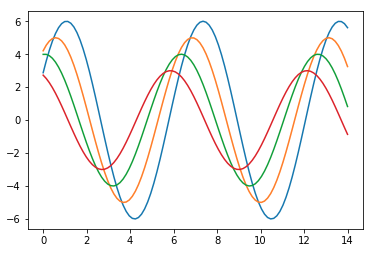To change the same plot to Seaborn defaults, use the set() function −

Example

import numpy as np
from matplotlib import pyplot as plt
def sinplot(flip = 1):
x = np.linspace(0, 14, 100)
for i in range(1, 5):
plt.plot(x, np.sin(x + i * .5) * (7 - i) * flip)
import seaborn as sb
sb.set()
sinplot()
plt.show()

Output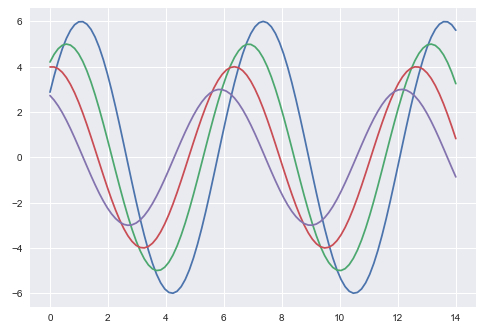The above two figures show the difference in the default Matplotlib and Seaborn plots. The representation of data is same, but the representation style varies in both.

Basically, Seaborn splits the Matplotlib parameters into two groups−

• Plot styles
• Plot scale

Seaborn Figure Styles

The interface for manipulating the styles is set_style(). Using this function you can set the theme of the plot. As per the latest updated version, below are the five themes available.

• Darkgrid
• Whitegrid
• Dark
• White
• Ticks

Let us try applying a theme from the above-mentioned list. The default theme of the plot will be darkgrid which we have seen in the previous example.

Example

import numpy as np
from matplotlib import pyplot as plt
def sinplot(flip=1):
x = np.linspace(0, 14, 100)
for i in range(1, 5):
plt.plot(x, np.sin(x + i * .5) * (7 - i) * flip)
import seaborn as sb
sb.set_style("whitegrid")
sinplot()
plt.show()

Output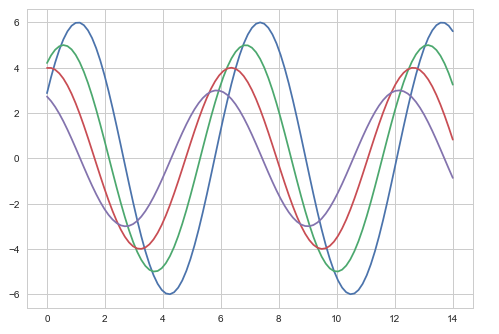The difference between the above two plots is the background color

Removing Axes Spines

In the white and ticks themes, we can remove the top and right axis spines using the despine() function.

Example

import numpy as np
from matplotlib import pyplot as plt
def sinplot(flip=1):
x = np.linspace(0, 14, 100)
for i in range(1, 5):
plt.plot(x, np.sin(x + i * .5) * (7 - i) * flip)
import seaborn as sb
sb.set_style("white")
sinplot()
sb.despine()
plt.show()

Output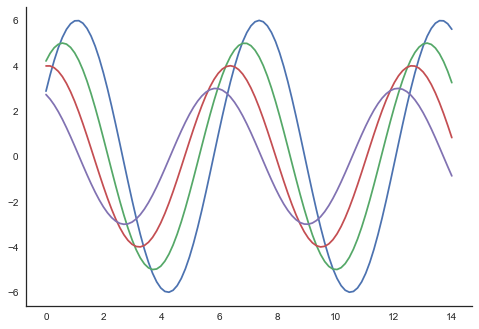In the regular plots, we use left and bottom axes only. Using the despine() function, we can avoid the unnecessary right and top axes spines, which is not supported in Matplotlib.

Overriding the Elements

If you want to customize the Seaborn styles, you can pass a dictionary of parameters to the set_style() function. Parameters available are viewed using axes_style() function.

Example

import seaborn as sb
print sb.axes_style

Output

{'axes.axisbelow'     : False,
'axes.edgecolor'      : 'white',
'axes.facecolor'      : '#EAEAF2',
'axes.grid'           : True,
'axes.labelcolor'     : '.15',
'axes.linewidth'      : 0.0,
'figure.facecolor'    : 'white',
'font.family'         : [u'sans-serif'],
'font.sans-serif'     : [u'Arial', u'Liberation
Sans', u'Bitstream Vera Sans', u'sans-serif'],
'grid.color'          : 'white',
'grid.linestyle'      : u'-',
'image.cmap'          : u'Greys',
'legend.frameon'      : False,
'legend.numpoints'    : 1,
'legend.scatterpoints': 1,
'lines.solid_capstyle': u'round',
'text.color'          : '.15',
'xtick.color'         : '.15',
'xtick.direction'     : u'out',
'xtick.major.size'    : 0.0,
'xtick.minor.size'    : 0.0,
'ytick.color'         : '.15',
'ytick.direction'     : u'out',
'ytick.major.size'    : 0.0,
'ytick.minor.size'    : 0.0}

Altering the values of any of the parameter will alter the plot style.

Example

import numpy as np
from matplotlib import pyplot as plt
def sinplot(flip=1):
x = np.linspace(0, 14, 100)
for i in range(1, 5):
plt.plot(x, np.sin(x + i * .5) * (7 - i) * flip)
import seaborn as sb
sb.set_style("darkgrid", {'axes.axisbelow': False})
sinplot()
sb.despine()
plt.show()

Output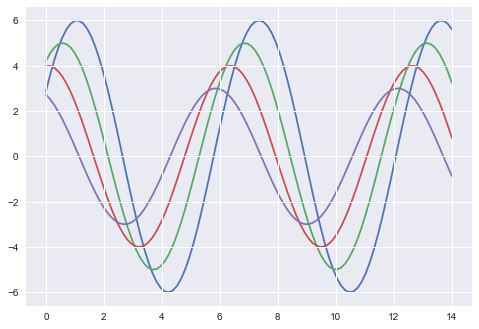Scaling Plot Elements

We also have control on the plot elements and can control the scale of plot using the set_context() function. We have four preset templates for contexts, based on relative size, the contexts are named as follows

• Paper
• Notebook
• Talk
• Poster

By default, context is set to notebook; and was used in the plots above.

Example

import numpy as np
from matplotlib import pyplot as plt
def sinplot(flip = 1):
x = np.linspace(0, 14, 100)
for i in range(1, 5):
plt.plot(x, np.sin(x + i * .5) * (7 - i) * flip)
import seaborn as sb
sb.set_style("darkgrid", {'axes.axisbelow': False})
sinplot()
sb.despine()
plt.show()

Output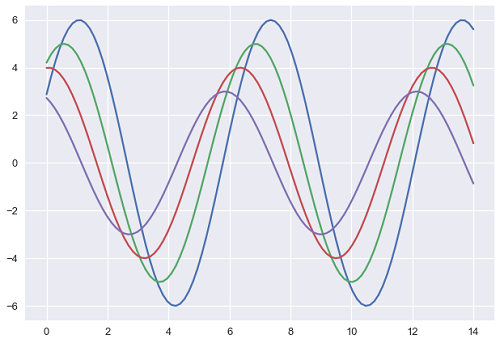The output size of the actual plot is bigger in size when compared to the above plots.

Note − Due to scaling of images on our web page, you might miss the actual difference in our example plots.

Useful Video Courses

Video

Data Visualization using MatPlotLib & Seaborn

11 Lectures 4 hours

Video

Seaborn with Python

11 Lectures 2.5 hours